### Home > CALC > Chapter 12 > Lesson 12.2.1 > Problem12-70

12-70.
1. Consider the infinite series below. For each, decide if it converges or diverges and justify your conclusion. State which test you used. Homework Help ✎

1.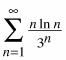2.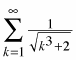3.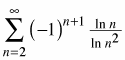4.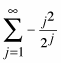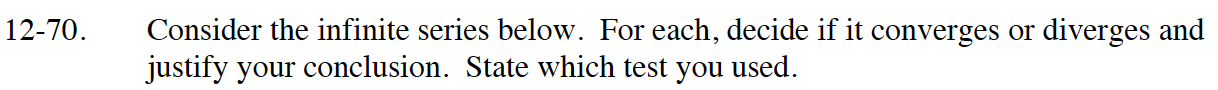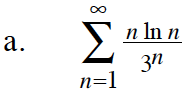Use the Ratio Test.

$\lim_{n\to\infty}\Bigg|\frac{\frac{(n+1)\ln(n+1)}{3^{n+1}}}{\frac{n\ln(n)}{3^n}}\Bigg|$

$\lim_{n\to\infty}\Big|\frac{(n+1)\ln(n+1)}{3n\ln(n)}\Big|=?$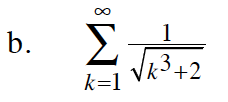Compare this series to:

$\sum_{n=1}^\infty \frac{1}{n^{3/2}}$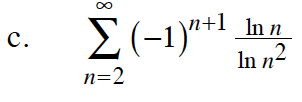This is an alternating series, so what is

$\lim_{n\to\infty}\frac{\ln(n)}{\ln(n^2)}=?$

$\ln(n^2)=2\ln(n)$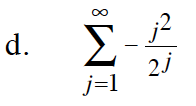Use the Ratio Test.